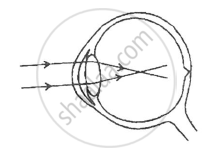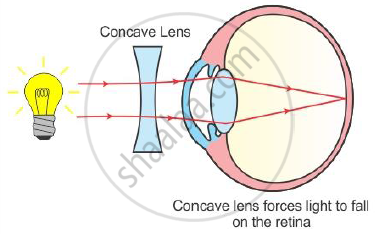Share

# Given below is a diagram depicting a defect of the human eye, study the same and then answer the questions that follow - ICSE Class 10 - Biology

ConceptEar: Parts and Functions of the Ear

#### Question

Given below is a diagram depicting a defect of the human eye, study the same and then answer the questions that follow:(i) Name the defect shown in the diagram.
(ii) What are the two possible that cause this defect?
(iii) Name the type of lens used to correct this defect.
(iv) With the help of a diagram show how the defect shown above is rectified using a suitable lens.

#### Solution

(i) Myopia
(ii) Two possible reasons are

• Eye ball is lengthened from front to back.
• Lens is too curved.

(iii) Concave lens

(iv)Is there an error in this question or solution?

#### APPEARS IN

2015-2016 (March) (with solutions)
Question 1.6 | 5.00 marks
Solution Given below is a diagram depicting a defect of the human eye, study the same and then answer the questions that follow Concept: Ear: Parts and Functions of the Ear.
S